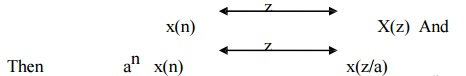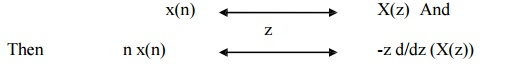Home | | Discrete Time Systems and Signal Processing | Properties of Z Transform (ZT)

# Properties of Z Transform (ZT)

1) Linearity 2) Time shifting 3) Scaling in z domain 4) Time reversal Property 5) Differentiation in z domain 6) Convolution Theorem 7) Correlation Property 8) Initial value Theorem 9) Final value Theorem

PROPERTIES OF Z TRANSFORM (ZT)

1) Linearity

The linearity property states that if zz Transform of linear     combination of two or more signals is equal to the same linear combination of z transform of individual signals.

2) Time shifting

The Time shifting property states that if z x(n)Thus shifting the sequence circularly by „k  samples is equivalent to multiplying its z transform by z –k

3) Scaling in z domain

This property states that ifThus scaling in z transform is equivalent to multiplying by an in time domain.

4) Time reversal Property

The Time reversal property states that if zIt means that if the sequence is folded it is equivalent to replacing z by z-1 in z domain.

5) Differentiation in z domain

The Differentiation property states that if z6) Convolution Theorem

The Circular property states that if zConvolution of two sequences in time domain corresponds to multiplication of its Z transform sequence in frequency domain.

7) Correlation Property

The Correlation of two sequences states that if z8) Initial value Theorem

Initial value theorem states that if z9) Final value Theorem

Final value theorem states that if zStudy Material, Lecturing Notes, Assignment, Reference, Wiki description explanation, brief detail
Digital Signal Processing : Signals and System : Properties of Z Transform (ZT) |